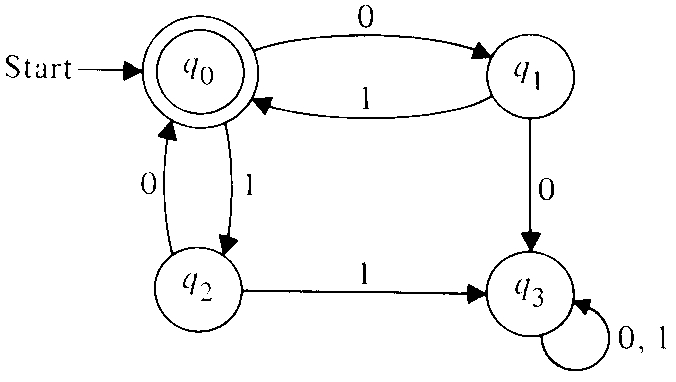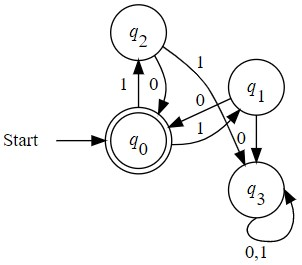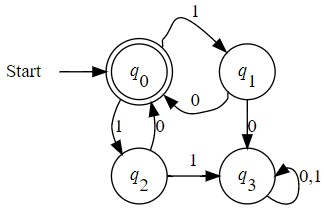# How to align nodes using a group attribute?

I tried to create graph from hereand want to get the exact picture, but can’t vertically align nodes and get nice curve in `q3->q3`.
My result is:How fix it?
My code:

``````digraph {
layout=dot
rankdir = LR

node [shape=circle]

Start [shape=plaintext group=g1]
q0 [shape = doublecircle label=<<I>q</I><SUB>0</SUB>> group=g1]
q1 [label=<<I>q</I><SUB>1</SUB>> group=g1]
q2 [label=<<I>q</I><SUB>2</SUB>> group=g2]
q3 [label=<<I>q</I><SUB>3</SUB>> group=g2]

Start -> q0
q0 -> q1 [label="1"]
q1 -> q0 [label="0"]
q1 -> q3 [label="0"]
q3:s -> q3:e [label="0,1"]
q0 -> q2 [label="1"]
q2 -> q0 [label="0"]
q2 -> q3 [label="1"]

{rank = same; q2;q0;}
{rank = same; q1;q3;}
}
``````

editor

Another variant:

``````digraph {
layout=dot
ranksep=0.2;

node [shape=circle]

Start [shape=plaintext group=g1]
q0 [shape = doublecircle label=<<I>q</I><SUB>0</SUB>> group=g1]
q1 [label=<<I>q</I><SUB>1</SUB>> group=g1]
q2 [label=<<I>q</I><SUB>2</SUB>> group=g2]
q3 [label=<<I>q</I><SUB>3</SUB>> group=g2]

Start -> q0
q0:ne -> q1:nw [label="1"]
q1:sw -> q0:se [label="0"]
q1 -> q3 [label="0"]
q3:se -> q3:e [label="0,1";]
q0 -> q2 [label="1"]
q2 -> q0 [label="0"]
q2 -> q3 [label="1"; constraint=false]

{rank=same; Start; q0; q1}
{rank=same; q2; q3}

//hack starting
//invisnode [shape=point, width=0]
edge [style=invis]
q0 -> q2
//q0 -> invisnode -> q1
}
``````

Result:If you figure it out, I’d love to hear your understanding of the “group” attribute. I was struggling to write some docs for it up on https://graphviz.org/docs/attrs/group/ the other day.

Unfortunately, the group attribute does pretty much what the documentation says. If the head and tail nodes of an edge have the same group value, dot will try to make the edge more vertical and try harder to avoid edge crossings. It only has effect on non-flat edges, so for this to have some effect in the second variant, the q0 and q2 would need the same group, as would q1 and q3. As a simple example, in the dot layout of the graph

``````digraph {
a [group=1]
c // [group=1]
a -> {c d}
b -> b1 -> c
b -> b2 -> d
a -> a2 -> d
}
``````

the edge a->c curves and has a crossing, but if you unquote the attribute on node c and layout the graph again, you’ll see that the edge a->c is straight, short, vertical and has no crossings.

As with various of the attributes, if used too much, the constraints can begin to conflict with each other and give unexpected results.

1 Like

I’m a little surprised your first attempt didn’t work. If you remove the rankdir statement, the result looks good. Setting rankdir=LR should just take that layout and rotate it 90 degrees. It sounds like a bug.

If you are not just exploring the group attribute and want a dot layout closer to the original, start with your second attempt and

1 Remove the group attributes, most of the compass point attributes, and the hack at the end
2 Add more space using the ranksep and nodesep attributes
3 Set the margin and minimum width of Start to 0
4 Use xlabels for the edges on q0

The items in 1 are not necessary; 2 helps with the flat edges between q0 and q1; 3 removes the space between the Start node and its edge. As for 4, edge labels are implemented as pseudo-nodes. This usually works well, providing the needed space, etc. But sometimes they can cause undesirable artifacts. In your case, you wanted arced edges on q0, but the edge labels caused some of the edges to be line segments. So you added compass points on the edges between q0 and q1, and needed an invisible edge from q0 to q2. Using xlabels causes the edges to be drawn first, often as you want them, and the labels are added afterwards.

The following graph should be close to what you want.

``````digraph {
ranksep=1;
nodesep=0.5;

node [shape=circle]

Start [margin=0 width=0 shape=plaintext]
q0 [shape = doublecircle label=<<I>q</I><SUB>0</SUB>>]
q1 [label=<<I>q</I><SUB>1</SUB>>]
q2 [label=<<I>q</I><SUB>2</SUB>>]
q3 [label=<<I>q</I><SUB>3</SUB>>]

Start -> q0
q1 -> q0 [xlabel="1"]
q0 -> q1 [xlabel="0"]
q1 -> q3 [label=" 0"]
q3:se -> q3:e [label=" 0,1"]
q2 -> q0 [xlabel="0 "]
q0 -> q2 [xlabel="1 "]
q2 -> q3 [label="1"]

{rank=same; Start; q0; q1}
{rank=same; q2; q3}
}
``````
1 Like

Thank you for the detailed explanation!